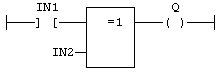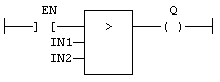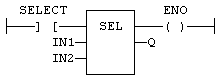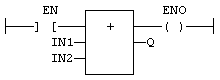A Ladder Diagram is a list of rungs. Each rung represents a boolean data flow from a power rail on the left to a power rail on the right. The left power rail represents the TRUE state. The data flow must be understood from the left to the right. Each symbol connected to the rung either changes the rung state or performs an operation. Below are possible graphic items to be entered in LD diagrams:

Power Rails
Contacts and Coils
Operations, Functions and Function blocks, represented by rectangular blocks
Labels and Jumps
Use of ST instructions in graphic languages

Use of the "EN" input and the "ENO" output for blocks

The rung state in a LD diagram is always boolean. Blocks are connected to the rung with their first input and output. This implies that special "EN" and "ENO" input and output are added to the block if its first input or output is not boolean.

The "EN" input is a condition. It means that the operation represented by the block is not performed if the rung state (EN) is FALSE. The "ENO" output always represents the sane status as the "EN" input: the rung state is not modified by a block having an ENO output.

Below is the example of the "XOR" block, having boolean inputs and outputs, and requiring no EN or ENO pin:

(* First input is the rung. The rung is the output *)Below is the example of the ">" (greater than) block, having non boolean inputs and a boolean output. This block has an "EN" input in LD language:

(* The comparison is executed only if EN is TRUE *)Below is the example of the "SEL" function, having a first boolean input, but an integer output. This block has an "ENO" output in LD language:

(* the input rung is the selector *)
(* ENO has the same value as SELECT *)Finally, below is the example of an addition, having only numerical arguments. This block has both "EN" and "ENO" pins in LD language:

(* The addition is executed only if EN is TRUE *)
(* ENO is equal to EN *)